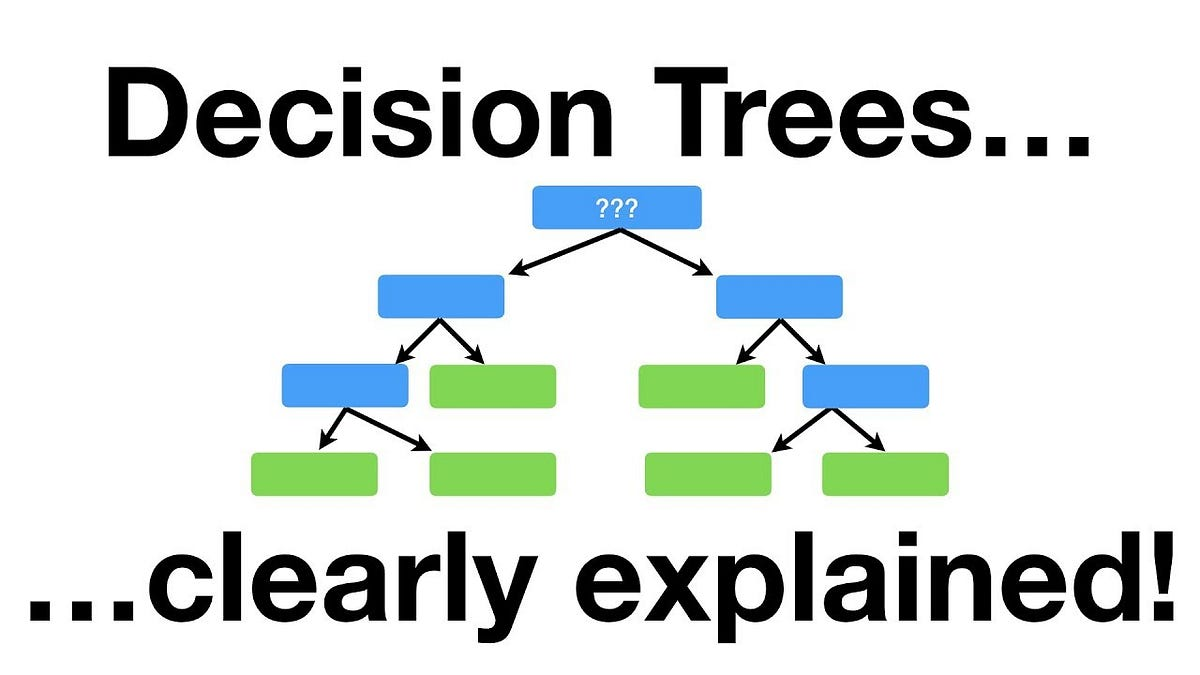# Decision Tree Classification in Python: Everything you need to knowWhat is Decision Tree? A decision tree is a decision support tool that uses a tree-like graph or model of decisions and their possible consequences, including chance event outcomes, resource costs, and utility. It is one way to display an algorithm that only contains conditional control statements.

## What is Decision Tree?

A decision tree is a decision support tool that uses a tree-like graph or model of decisions and their possible consequences, including chance event outcomes, resource costs, and utility. It is one way to display an algorithm that only contains conditional control statements.

Decision Trees (DTs) are a non-parametric supervised learning method used for both classification and regression. Decision trees learn from data to approximate a sine curve with a set of if-then-else decision rules. The deeper the tree, the more complex the decision rules, and the fitter the model. The decision tree builds classification or regression models in the form of a tree structure, hence called CART (Classification and Regression Trees). It breaks down a data set into smaller and smaller subsets building along an associated decision tree at the same time. The final result is a tree with decision nodes and leaf nodes. A decision node has two or more branches. The leaf node represents a classification or decision. The topmost decision node in a tree which corresponds to the best predictor called the root node. Decision trees can handle both categorical and numerical data.

## When is Decision Tree Used?

1. When the user has and objective and he is trying to achieve max profit, optimized cost, etc.
2. When there are several courses of action like the menu system in an ATM machine, Customer Support calling menu, etc.
3. Uncertainty concerning which outcome will actually happen.

## How to Make a Decision Tree?

Step 1

Calculate the entropy of the target.

Step 2

The dataset is then split into different attributes. The entropy for each branch is calculated. Then it is added proportionally, to get total entropy for the split. The resulting entropy is subtracted from the entropy before the split. The result is the Information Gain or decrease in entropy.

## Data Science With Python Training | Python Data Science Course | Intellipaat

🔵 Intellipaat Data Science with Python course: https://intellipaat.com/python-for-data-science-training/In this Data Science With Python Training video, you...

## Applied Data Analysis in Python Machine Learning and Data Science | Scikit-Learn

Applied Data Analysis in Python Machine learning and Data science, we will investigate the use of scikit-learn for machine learning to discover things about whatever data may come across your desk.

## Data Science Projects | Data Science | Machine Learning | Python

Practice your skills in Data Science with Python, by learning and then trying all these hands-on, interactive projects, that I have posted for you.

## Data Science Projects | Data Science | Machine Learning | Python

Practice your skills in Data Science with Python, by learning and then trying all these hands-on, interactive projects, that I have posted for you.

## Data Science Projects | Data Science | Machine Learning | Python

Practice your skills in Data Science with Python, by learning and then trying all these hands-on, interactive projects, that I have posted for you.